# 目录

### 第一步，准备

1. 初始化参数：args = parse_args() 采用的是Python的argparse
主要有–net_name，–gpu，–cfg等（在cfg中只是修改了几个参数，其他大部分参数在congig.py中，涉及到训练整个网络）。
2. cfg_from_file(args.cfg_file) 这里便是代用config中的函数cfg_from_file来读取前面cfg文件中的参数，同时调用_merge_a_into_b函数把所有的参数整合，其中__C = edict() cfg = __C cfg是一个词典（edict）数据结构。
3. faster rcnn采用的是多进程，mp_queue是进程间用于通讯的数据结构
import multiprocessing as mp
mp_queue = mp.Queue()

### 第二步，Stage 1 RPN, init from ImageNet model

cfg.TRAIN.SNAPSHOT_INFIX = 'stage1'
mp_kwargs = dict(
queue=mp_queue,
imdb_name=args.imdb_name,
init_model=args.pretrained_model,
solver=solvers,
max_iters=max_iters,
cfg=cfg)
p = mp.Process(target=train_rpn, kwargs=mp_kwargs)
p.start()
rpn_stage1_out = mp_queue.get()
p.join()

#### 1.在config参数的基础上改动参数，以适合当前任务，主要有

cfg.TRAIN.HAS_RPN = True
cfg.TRAIN.BBOX_REG = False  # applies only to Fast R-CNN bbox regression
cfg.TRAIN.PROPOSAL_METHOD = 'gt'

#### 3. 准备roidb和imdb

{
year:’2007’
image _set:’trainval’
devkit _path:’data/VOCdevkit2007’
data _path:’data /VOCdevkit2007/VOC2007’
classes:(…)_如果想要训练自己的数据，需要修改这里_
class _to _ind:{…} _一个将类名转换成下标的字典 _  建立索引0,1,2....
image _ext:’.jpg’
image _index: [‘000001’,’000003’,……]_根据trainval.txt获取到的image索引_
roidb _handler: <Method gt_roidb >
salt:  <Object uuid >
comp _id:’comp4’
config:{…}
}

imdb.set_proposal_method(cfg.TRAIN.PROPOSAL_METHOD)

• boxes 一个二维数组，每一行存储 xmin ymin xmax ymax
• gt _classes存储了每个box所对应的类索引(类数组在初始化函数中声明)
• gt _overlap是一个二维数组，共有num _classes(即类的个数)行，每一行对应的box的类索引处值为1，其余皆为0，后来被转成了稀疏矩阵
• seg _areas存储着某个box的面积
• flipped 为false 代表该图片还未被翻转(后来在train.py里会将翻转的图片加进去，用该变量用于区分

return roidb，imdb

#### 5.正式开始训练

model_paths = train_net(solver, roidb, output_dir,
pretrained_model=init_model,
max_iters=max_iters)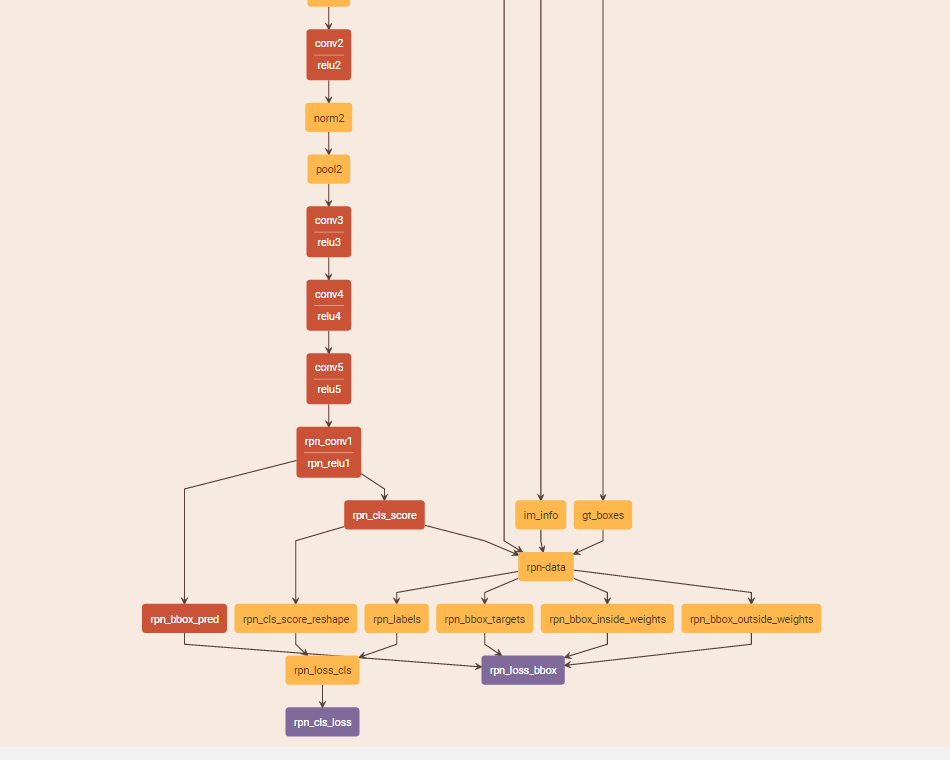#### 6.保存最后得到的权重参数

rpn_stage1_out = mp_queue.get()

### 第三步，Stage 1 RPN, generate proposals

mp_kwargs = dict(
queue=mp_queue,
imdb_name=args.imdb_name,
rpn_model_path=str(rpn_stage1_out['model_path']),
cfg=cfg,
rpn_test_prototxt=rpn_test_prototxt)
p = mp.Process(target=rpn_generate, kwargs=mp_kwargs)
p.start()
rpn_stage1_out['proposal_path'] = mp_queue.get()['proposal_path']
p.join()

#### 1.关注rpn_generate函数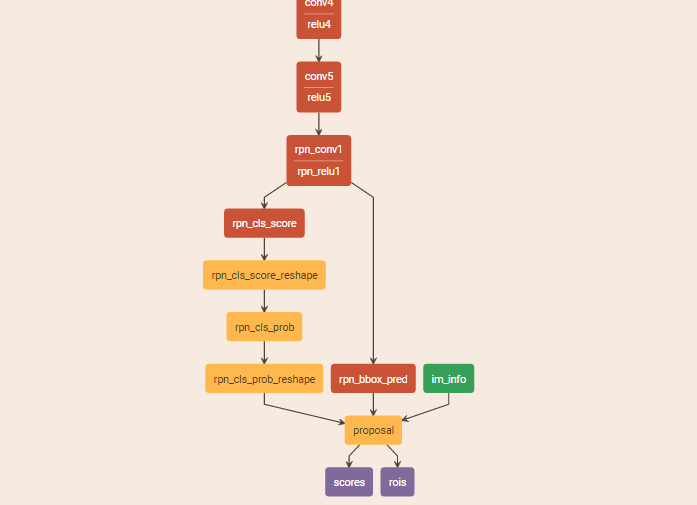boxes = blobs_out['rois'][:, 1:].copy() / scale
scores = blobs_out['scores'].copy()
return boxes, scores

#### 2.保存得到的proposal文件

queue.put({'proposal_path': rpn_proposals_path})
rpn_stage1_out['proposal_path'] = mp_queue.get()['proposal_path']

### 第四步，Stage 1 Fast R-CNN using RPN proposals, init from ImageNet model

cfg.TRAIN.SNAPSHOT_INFIX = 'stage1'
mp_kwargs = dict(
queue=mp_queue,
imdb_name=args.imdb_name,
init_model=args.pretrained_model,
solver=solvers,
max_iters=max_iters,
cfg=cfg,
rpn_file=rpn_stage1_out['proposal_path'])
p = mp.Process(target=train_fast_rcnn, kwargs=mp_kwargs)
p.start()
fast_rcnn_stage1_out = mp_queue.get()
p.join()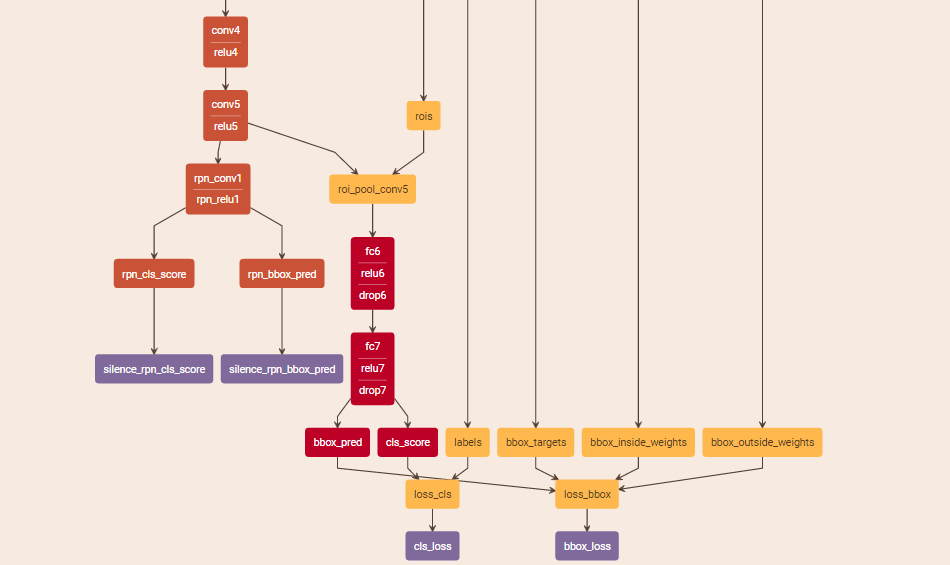### 第五步，Stage 2 RPN, init from stage 1 Fast R-CNN model

cfg.TRAIN.SNAPSHOT_INFIX = 'stage2'
mp_kwargs = dict(
queue=mp_queue,
imdb_name=args.imdb_name,
init_model=str(fast_rcnn_stage1_out['model_path']),
solver=solvers,
max_iters=max_iters,
cfg=cfg)
p = mp.Process(target=train_rpn, kwargs=mp_kwargs)
rpn_stage2_out = mp_queue.get()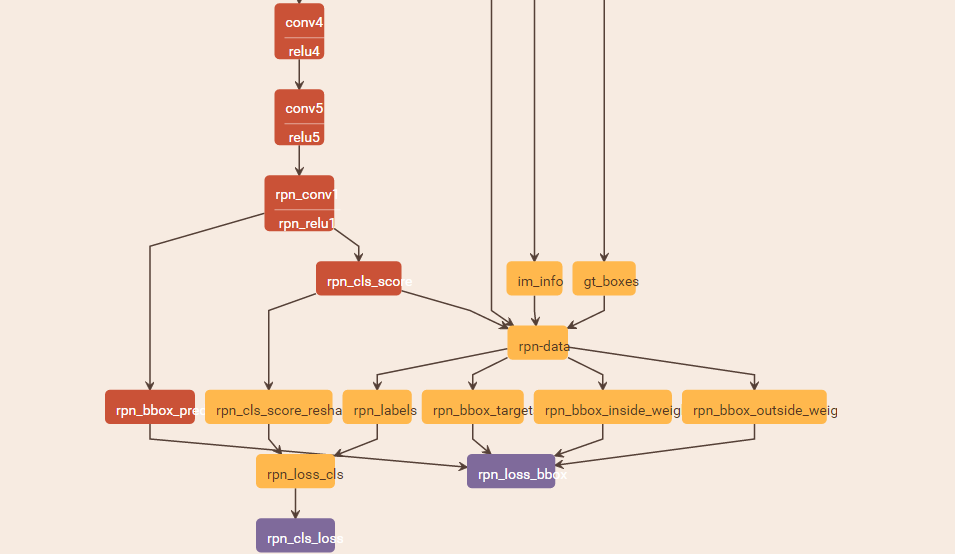### 第六步，Stage 2 RPN, generate proposals

mp_kwargs = dict(
queue=mp_queue,
imdb_name=args.imdb_name,
rpn_model_path=str(rpn_stage2_out['model_path']),
cfg=cfg,
rpn_test_prototxt=rpn_test_prototxt)
p = mp.Process(target=rpn_generate, kwargs=mp_kwargs)
p.start()
rpn_stage2_out['proposal_path'] = mp_queue.get()['proposal_path']
p.join()### 第七步，Stage 2 Fast R-CNN, init from stage 2 RPN R-CNN model

cfg.TRAIN.SNAPSHOT_INFIX = 'stage2'
mp_kwargs = dict(
queue=mp_queue,
imdb_name=args.imdb_name,
init_model=str(rpn_stage2_out['model_path']),
solver=solvers,
max_iters=max_iters,
cfg=cfg,
rpn_file=rpn_stage2_out['proposal_path'])
p = mp.Process(target=train_fast_rcnn, kwargs=mp_kwargs)
p.start()
fast_rcnn_stage2_out = mp_queue.get()
p.join()### 第八步，输出最后模型

final_path = os.path.join(
os.path.dirname(fast_rcnn_stage2_out['model_path']),
args.net_name + '_faster_rcnn_final.caffemodel')
print 'cp {} -> {}'.format(
fast_rcnn_stage2_out['model_path'], final_path)
shutil.copy(fast_rcnn_stage2_out['model_path'], final_path)
print 'Final model: {}'.format(final_path)

### AnchorTargetLayer和ProposalLayer

class AnchorTargetLayer(caffe.Layer)

anchor_scales = layer_params.get('scales', (8, 16, 32))
self._anchors = generate_anchors(scales=np.array(anchor_scales))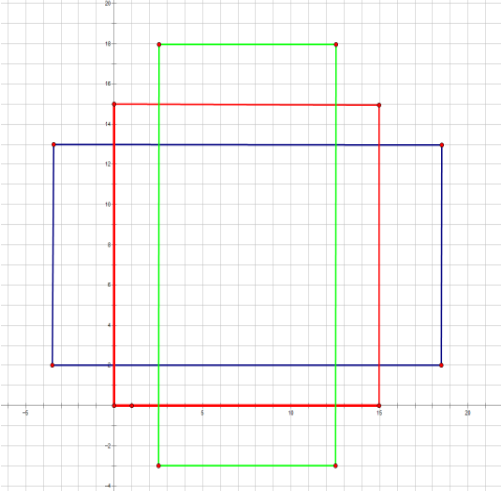_scale_enum()部分，生成三种尺寸的anchor，以_ratio_enum()部分生成的anchor[0 0 15 15]为例，扩展了三种尺度 128*128,256*256,512*512，如下图所示：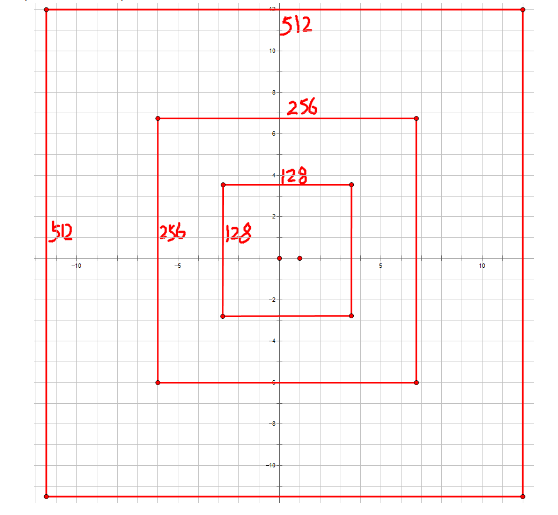# Algorithm:
# for each (H, W) location i
#   generate A anchor boxes centered on cell i
#   apply predicted bbox deltas at cell i to each of the A anchors
# clip predicted boxes to image
# remove predicted boxes with either height or width < threshold
# sort all (proposal, score) pairs by score from highest to lowest
# take top pre_nms_topN proposals before NMS
# apply NMS with threshold 0.7 to remaining proposals
# take after_nms_topN proposals after NMS
# return the top proposals (-> RoIs top, scores top)

### 代码文件夹说明

#### tools

• _init_paths.py :用来初始化路径的，也就是之后的路径会join（path，*）
• compress_net.py：用来压缩参数的，使用了SVD来进行压缩，这里可以发现，作者对于fc6层和fc7层进行了压缩，也就是两个全连接层。
• demo.py ：通常，我们会直接调用这个函数，如果要测试自己的模型和数据，这里需要修改。这里调用了fast_rcnn中的test、config、nums_wrapper函数。vis_detections用来做检测，parse_args用来进行参数设置，以及damo和主函数。
• eval_recall.py：评估函数
• reval.py：re-evaluate，这里调用了fast_rcnn以及dataset中的函数。其中，from_mats函数和from_dets函数分别loadmat文件和pkl文件。
• rpn_genetate.py：这个函数调用了rpn中的genetate函数，之后我们会对rpn层做具体的介绍。这里，主要是一个封装调用的过程，我们在这里调用配置的参数、设置rpn的test参数，以及输入输出等操作。
• test_net.py：测试fast rcnn网络。主要就是一些参数配置。
• train_faster_rcnn_alt_opt.py：训练faster rcnn网络使用交替的训练，这里就是根据faster rcnn文章中的具体实现。可以在主函数中看到，其包括的步骤为：
• RPN 1，使用imagenet model进行初始化参数，生成proposal，这里存储在mp_kwargs
• fast rcnn 1，使用 imagenet model 进行初始化参数，使用刚刚生成的proposal进行fast rcnn的训练
• RPN 2使用 fast rcnn 中的参数进行初始化（这里要注意哦），并生成proposal
• fast rcnn 2，使用RPN 2 中的 model进行初始化参数
• 值得注意的是：在我们训练时，我们可以在get_solvers中的max_iters中设置迭代次数，在不确定网络是否可以调通时，减少迭代次数可以减少测试时间。
• 我们在训练faster rcnn网络时，就是调用这个文件训练的
• train_net.py：使用fast rcnn，训练自己数据集的网络模型
• train_svms.py：使用最原始的RCNN网络训练post-hoc SVMs

#### RPN

• generate_anchors.py: 生成多尺度和多比例的锚点。这里由generate_anthors函数主要完成，可以看到，使用了 3 个尺度( 128, 256, and 512)以及 3 个比例(1:1,1:2,2:1)。一个锚点由w, h, x_ctr, y_ctr固定，也就是宽、高、x center和y center固定。
• proposal_layer.py:这个函数是用来将RPN的输出转变为object proposals的。作者新增了ProposalLayer类，这个类中，重新了set_up和forward函数，其中forward实现了：生成锚点box、对于每个锚点提供box的参数细节、将预测框切成图像、删除宽、高小于阈值的框、将所有的(proposal, score) 对排序、获取 pre_nms_topN proposals、获取NMS 、获取 after_nms_topN proposals。（注：NMS，nonmaximum suppression，非极大值抑制）
• anchor_target_layer.py:生成每个锚点的训练目标和标签，将其分类为1 (object), 0 (not object) ， -1 (ignore).当label>0，也就是有object时，将会进行box的回归。其中，forward函数功能：在每一个cell中，生成9个锚点，提供这9个锚点的细节信息，过滤掉超过图像的锚点，测量同GT的overlap。
• proposal_target_layer.py:对于每一个object proposal 生成训练的目标和标签，分类标签从0-k，对于标签>0的box进行回归。（注意，同anchor_target_layer.py不同，两者一个是生成anchor，一个是生成proposal）
• generate.py:使用一个rpn生成object proposals。

#### nms

lib/nms文件夹下是非极大值抑制，这部分大家应该已经非常熟悉了，其Python版本的核心函数为py_cpu_nms.py，具体实现以及注释如下：

def py_cpu_nms(dets, thresh):
"""Pure Python NMS baseline."""
#x1、y1、x2、y2、以及score赋值
x1 = dets[:, 0]
y1 = dets[:, 1]
x2 = dets[:, 2]
y2 = dets[:, 3]
scores = dets[:, 4]

#每一个op的面积
areas = (x2 - x1 + 1) * (y2 - y1 + 1)
#order是按照score排序的
order = scores.argsort()[::-1]

keep = []
while order.size > 0:
i = order
keep.append(i)
xx1 = np.maximum(x1[i], x1[order[1:]])
yy1 = np.maximum(y1[i], y1[order[1:]])
xx2 = np.minimum(x2[i], x2[order[1:]])
yy2 = np.minimum(y2[i], y2[order[1:]])

#计算相交的面积
w = np.maximum(0.0, xx2 - xx1 + 1)
h = np.maximum(0.0, yy2 - yy1 + 1)
inter = w * h
#计算：重叠面积/（面积1+面积2-重叠面积）
ovr = inter / (areas[i] + areas[order[1:]] - inter)

inds = np.where(ovr <= thresh)
order = order[inds + 1]

### 参考

 http://www.cnblogs.com/CarryPotMan/p/5390336.html
 http://blog.csdn.net/u010668907/article/category/6237110
 http://blog.csdn.net/sunyiyou9/article/category/6269359
 http://blog.csdn.net/bailufeiyan/article/details/50749694

### 原文地址：

http://blog.csdn.net/u011956147/article/details/53053381